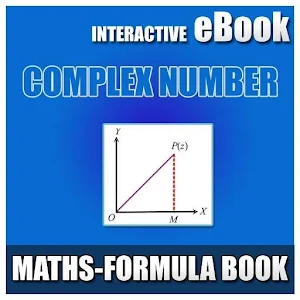# COMPLEX NUMBER-FORMULA BOOK - Lumos Educational App Store51
Price -NA
\$A

#### DESCRIPTION:

Complex Number Interactive e-Book for IIT-JEE & other Competitive Exams. Board: CBSE, GSEB, ICSE, ISC, International Board, Cambridge Class: 11th - 12th Students, Engineering Students and Medical Students. Maths (AIEEE, IIT-JEE Main) Complex Number Interactive e-Book Education application Topic: Basic Concepts of Complex Number Real and Imaginary Parts of a Complex Number Algebraic Operations with Complex Numbers Properties of algebraic operations on complex numbers Equality of Two Complex Numbers Conjugate of a complex number Modulus of a Complex Number Properties of Modulus

#### OVERVIEW:

COMPLEX NUMBER-FORMULA BOOK is a free educational mobile app By Learn with Quiz.It helps students in grades HS practice the following standards HSN.CN.A.1,HSN.CN.A.2,HSN.CN.A.3.

This page not only allows students and teachers download COMPLEX NUMBER-FORMULA BOOK but also find engaging Sample Questions, Videos, Pins, Worksheets, Books related to the following topics.

1. HSN.CN.A.1 : Know there is a complex number i such that i2 = -1, and every complex number has the form a + bi with a and b real.

2. HSN.CN.A.2 : Use the relation i2 = -1 and the commutative, associative, and distributive properties to add, subtract, and multiply complex numbers..

3. HSN.CN.A.3 : Find the conjugate of a complex number; use conjugates to find moduli and quotients of complex numbers..

HS

#### STANDARDS:

HSN.CN.A.1
HSN.CN.A.2
HSN.CN.A.3
HSN.CN.B.4
HSN.CN.B.5
HSN.CN.B.6
HSN.CN.C.7
HSN.CN.C.8
HSN.CN.C.9

Developer: Learn with Quiz

Software Version: 0.1

Category: Education

### RELATED APPSEdSearch WebSearch